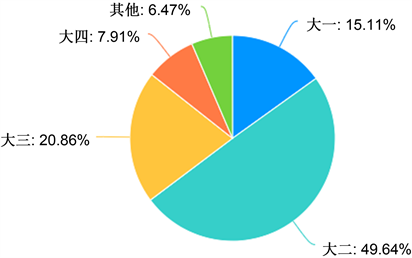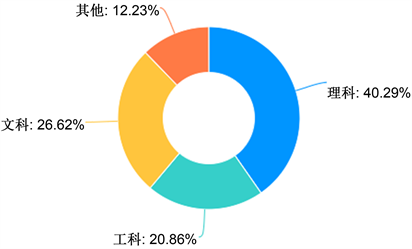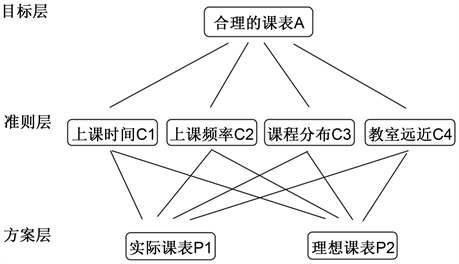﻿ 高校教务排课系统的应用研究

# 高校教务排课系统的应用研究Research on the System to Arrange Courses of Universities

Abstract: With the development of science and technology, the courses arrangement of colleges and universities is gradually changing from manual to automatic. We have done a research to analyze whether the arrangement of courses and universities is reasonable. Guided by the analytic hierarchy process and fuzzy comprehension evaluation method, we mainly studied the four factors concerning courses arrangement: course distribution, course time, distance of adjacent courses and capacity of classrooms. Firstly, we distributed questionnaires to college students to acquire general information of courses arrangement. After obtaining effective information, we exerted analytic hierarchy process and invited experts to score the four factors. We determined the weight and the value of the four factors. Then we took the statistics 1701 of Yangzhou University as an example to analyze whether the courses arrangement is reasonable. And then, we collected ten questionnaires from teachers to analyze the four factors. Finally, we used the fuzzy comprehension evaluation method to draw the conclusion that the teachers have a good evaluation on the courses arrangement. This article aims to improve the automatic courses arrangement system and provide more reasonable courses schedule for students and teachers. The conclusion shows that the courses schedule has nothing unreasonable. But there are still some shortcomings to improve. We also put forward some advice at the end of the article for you.

1. 引言

2. 以层次分析法对排课系统进行分析

2.1. 建立层次分析模型Figure 2. Professional distribution mapFigure 3. Analytic hierarchy model

2.2. 构造准则层对目标层的成对比较阵

$A=\left(\begin{array}{cccc}1.0& 1.5& 3.0& 1.8\\ 0.6& 1.0& 2.0& 1.2\\ 0.3& 0.5& 1.0& 0.6\\ 0.5& 0.8& 1.6& 1.0\end{array}\right)$

$\left(\begin{array}{cccc}0.45& 0.39& 0.39& 0.39\\ 0.25& 0.26& 0.26& 0.26\\ 0.125& 0.13& 0.13& 0.13\\ 0.21& 0.21& 0.21& 0.21\end{array}\right)$

$w=\left(\begin{array}{c}0.3913\\ 0.2609\\ 0.1304\\ 0.2173\end{array}\right)$

$Aw=\left(\begin{array}{c}1.5650\\ 1.0172\\ 0.5086\\ 0.8306\end{array}\right)$

$\lambda =\frac{1}{4}\left(\frac{1.5650}{0.3913}+\frac{1.0172}{0.2609}+\frac{0.5086}{0.1304}+\frac{0.8306}{0.2173}\right)=3.9049$

2.3. 确定准则层对目标层的权向量

${w}^{\left(2\right)}={\left(0.3913,\text{\hspace{0.17em}}0.2609,\text{\hspace{0.17em}}0.1304,\text{\hspace{0.17em}}0.2173\right)}^{\prime }$

2.4. 一致性检验

3. 以模糊评价法对排课系统进行分析

3.1. 模糊评价法基本步骤

3.1.1. 简介

3.1.2. 模糊综合评价法优缺点

3.1.3. 确定评价对象的因素集

3.1.4. 建立评价集

3.1.5. 建立模糊关系矩阵

3.1.6. 确定权重集

3.1.7. 模糊综合评判

$B=A\ast R=\left({a}_{1},\text{\hspace{0.17em}}{a}_{2},\text{\hspace{0.17em}}\cdots ,\text{\hspace{0.17em}}{a}_{n}\right)\left(\begin{array}{ccc}{r}_{11}& \cdots & {r}_{1m}\\ ⋮& \ddots & ⋮\\ {r}_{n1}& \cdots & {r}_{nm}\end{array}\right)$ “*”运算符表示将对应元素两两比较先取小，然后再在所在结

3.2. 问卷分析

$\left(\begin{array}{cccc}0.51& 0.15& 0.18& 0.16\\ 0.88& 0.12& 0.00& 0.00\\ 0.97& 0.00& 0.00& 0.03\\ 0.00& 0.00& 0.20& 0.80\end{array}\right)$

3.3. 模糊评价法结论

4. 本文结论

NOTES

*通讯作者。

 王鹏亮, 裴海瑞. 基于UML的高校机房排课系统的设计[J]. 计算机产品与流通, 2020(5): 178.

 刘光盛. 高校排课系统的设计与实现研究[J]. 科技风, 2020(5): 80.

 吕牡丹. 基于JSP技术的高校智能排课系统的设计[J]. 计算机产品与流通, 2019(7): 210.

 王庆鹏, 张升. 述论内蒙古师范大学教务排课情况[J]. 内蒙古教育, 2019(14): 10-13.

 武建新. 基于教务管理系统的高校最优化排课的研究[J]. 智库时代, 2018(39): 89+94.

 胡珊, 刘晶. 模糊综合评价法在产品设计方案决策中的应用[J]. 机械设计, 2020, 37(1): 135-139.

 何梅. 基于模糊综合评价法的本科教学评价[J]. 中国多媒体与网络教学学报(上旬刊), 2020(7): 34-36.

Top